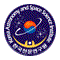# East Asian VLBI Network (EAVN)

## Sensitivity

When a target source is observed,a noise level σbl for each baseline can be expressed as(2)

where k is Boltzmann constant, η is quantization efficiency (~0.88), Tsys is system noise temperature, SEFD is system equivalent flux density, Ae is antenna effective aperture area (Ae = πηAD2 / 4 in which Ae and D are the aperture efficiency and antenna diameter, respectively), B is the bandwidth, and τ is on-source integration time. Note that for an integration time beyond 3 minutes (in the K band), the noise level expected by equation (2) cannot be attained because of the coherence loss due to the atmospheric fluctuation. Thus, for finding fringe within a coherence time, the integration time τ cannot be longer than 3 minutes. For VLBI observations, signal-to-noise ratio (S/N) of at least 5 and usually 7 is generally required for finding fringes.

A resultant image noise level σim can be expressed as(3)

If the array consists of identical antennas, an image noise levels can be expressed as(4)

where N is the number of antennas. Using the typical parameters shown in Table 7, baseline and image sensitivity values of EAVN are calculated as listed in Tables 8 and Table 9 (baseline and image sensitivities of KVN, VERA, and KaVA, as well as EAVN, are also shown for reference).

Table 7: Parameters of each telescope.
Station K band Q band
Tsys [K] ηA SEFD [Jy] Tsys [K] ηA SEFD [Jy]
KVN1000.613281500.61992
VERA1200.521102500.54395
Tianma600.5100660.5110
NRO45---2000.53655
Table 8: Baseline sensitivity of EAVN.
K bandQ band
KVNVERATianmaMRO45KVNVERATianmaMRO45
KVN6.17.71.7-9.113.62.25.2
VERA-9.72.1--20.23.27.8
Tianma-------1.2
Note: 1σ baseline sensitivity values are listed in unit of mJy, which assume an integration time of 120 seconds and a bandwidth of 256MHz for the calculation. In the case of narrower bandwidth of 15.625 KHz (for maser emission), sensitivities can be calculated by multiplying a factor of 128.

Figures 7 and 8 show the system noise temperature at Mizusawa and Ulsan, respectively. For Mizusawa, receiver noise temperatures are also plotted.

Note that the receiver temperature of VERA includes the temperature increase due to the feedome loss and the spill-over eﬀect. In Mizusawa, typical system temperature in the K-band is Tsys = 150 K in ﬁne weather of winter season, but sometimes rises above Tsys = 300 K in summer season. The system temperature at Iriki station shows a similar tendency to that in Mizusawa. In Ogasawara and Ishigakijima, typical system temperature is similar to that for summer in Mizusawa site, with typical optical depth of τ0 = 0.2 ∼ 0.3. The typical system temperature in the Q-band in Mizusawa is Tsys = 250 K in ﬁne weather of winter season, and Tsys = 300 − 400 K in summer season. The typical system temperature in Ogasawara and Ishigakijima in the Q-band is larger than that in Mizusawa also.

The typical system temperature in the K-band at all KVN stations is around 100 K in winter season. In summer season, it increases up to ∼ 300 K. In the Q-band, the typical system temperature is around 150 K in winter season and 250 K in summer season at Yonsei and Tamna. The system temperature of Ulsan in the Q-band is about 40 K lower than the other two KVN stations. This is mainly due to the diﬀerence in receiver noise temperature (see Table 4).

Table 9: Image sensitivity of EAVN.
ArrayNantNblK bandQ band
KVN33320480
VERA46360750
KaVA721155268
KaVA+Tianma8286085
KaVA+NRO45828-169
KaVA+Tianma+NRO45936-65
Note: N antand Nbl are the numbers of telescopes and baselines for each array. 1σ image sensitivity values are listed in unit of μJy, which assume an integration time of 4 hours and a total bandwidth of 256 MHz for the calculation. In the case of narrower bandwidth of 15.625 kHz (for maser emission), sensitivities can be calculated by multiplying a factor of 128.EAVN ( East Asia VLBI Network )